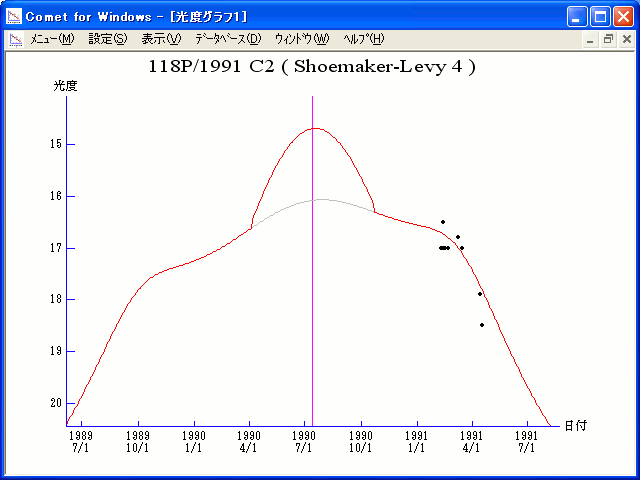# 118P/Shoemaker-Levy 4 (1990)###Magnitudes Graph

```        m1 =  8.2 + 5 log d + 18.0 log r  [    ,-100]  (             - 1990 Apr.  5)
m1 = -6.0 + 5 log d + 60.0 log r  [-100, 100]  (1990 Apr.  5 - 1990 Oct. 22)
m1 =  8.2 + 5 log d + 18.0 log r  [ 100,    ]  (1990 Oct. 22 -             )

* Gray curve is:  m1 = 8.2 + 5 log d + 18.0 log r
```##### The magnitudes graphs are made with Comet for Windows.